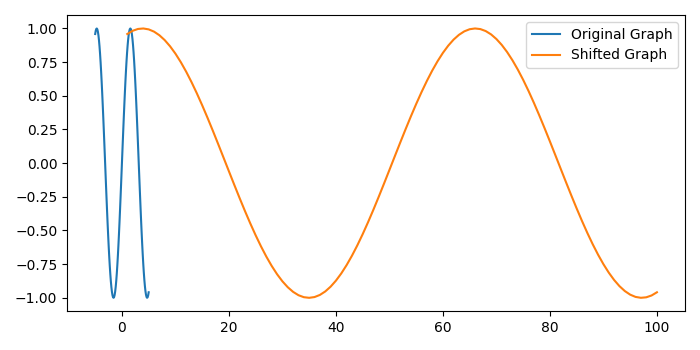# How to shift a graph along the X-axis in matplotlib?

To shift a graph along the X-axis in matplotlib, we can take the following steps −

• Set the figure size and adjust the padding between and around the subplots.
• Create x and y data points using numpy.
• Plot the x and y data points for the original curve.
• Plot the shifted graph, in the range of (1, 1+len(y)) with y data points.
• Place a legend on the figure.
• To display the figure, use show() method.

## Example

import numpy as np
import matplotlib.pyplot as plt

# Set the figure size
plt.rcParams["figure.figsize"] = [7.00, 3.50]
plt.rcParams["figure.autolayout"] = True

# x and y data points
x = np.linspace(-5, 5, 100)
y = np.sin(x)

# Original graph and shifted graph
plt.plot(x, y, label='Original Graph')
plt.plot(range(1, 1+len(y)), y, label='Shifted Graph')

# Place a legend
plt.legend(loc='upper right')

plt.show()

## Output

It will produce the following output